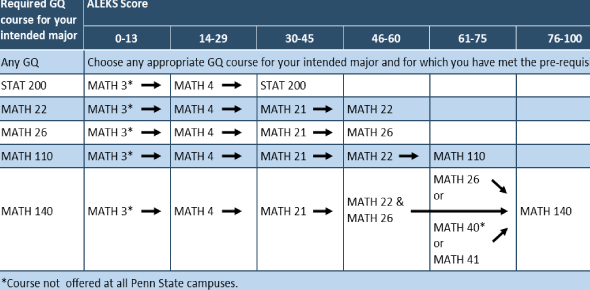# Math Assessment Topic 1

8 Questions | Total Attempts: 77SettingsTopic One: Place Value, Comparing numbers and decimals

• 1.
What is the value of the underlined digit in 10.596 ?
• A.

0.6

• B.

0.06

• C.

0.006

• D.

0.0006

• 2.
Which of the following is the word form of 7.24 ?
• A.

Seven and twenty-four tenths

• B.

Seven and twenty-four hundredths

• C.

Seven and two hundred four hundredths

• D.

Seven and two hundred forty thousandths

• 3.
What is the number eight million, three hundred thousand, fifty-three  in standard form ?
• A.

8,030,530

• B.

8,300,053

• C.

8,350,035

• D.

8,300,035

• 4.
What is 30,270,904 in expanded form ?
• A.

3,000,000 + 200,000 + 70,000 900 + 4

• B.

30,000,000 + 200,000 + 7,000 + 900 + 4

• C.

30,000,000 + 20,000 + 70,000 + 900 + 4

• D.

30,000,000 + 200,000 + 70,000 + 900 + 4

• 5.
Which of the following is true about the two numbers  A & B?               A) 4, 260,487      B) 4,260,480
• A.

A>B

• B.

A

• C.

A=B

• D.

None of the above

• 6.
What is the value of the underlined digit ?    12,864,125
• A.

600

• B.

6,000

• C.

60,000

• D.

600,000

• 7.
Which of the following is eighteen and sixteen hundredths written in standard form?
• A.

8.16

• B.

18.16

• C.

8.106

• D.

18.106

• 8.
What number is missing from the following numbers  ?    0.14  - 0.24 - 0.34 - 0.44 - 0.?? - 0.64
• A.

0.74

• B.

0.45

• C.

0.04

• D.

0.54

Related TopicsBack to top